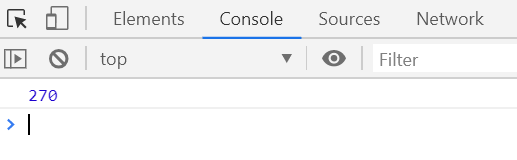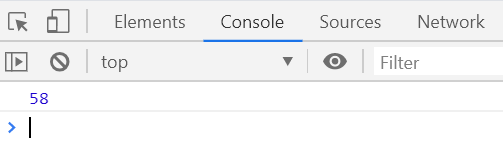# Underscore.js | _.partial() Function

The _.partial() function is used to apply partially a function by filling in any number of its arguments, without changing its dynamic value.

Syntax:

`_.partial(function, *arguments)`

Parameters: This function accept two parameters as mentioned above and described below:

• function: The function that need to be executed.
• arguments: This parameter needs to add some symbols between elements.

Return Value: This function returns the result of partially executed function.

Below examples illustrate the _.partial() Function in Underscore.js:
Example 1:

 ` ` `<``html``> ` ` `  `<``head``> ` `    ``<``script` `type``=``"text/javascript"` `src``= ` `"https://cdnjs.cloudflare.com/ajax/libs/underscore.js/1.9.1/underscore-min.js"``> ` `    `` ` ` ` ` `  `<``body``> ` `    ``<``script` `type``=``"text/javascript"``> ` ` `  `        ``var product = function (num1, num2) { ` `            ``return num1 * num2; ` `        ``}; ` ` `  `        ``prod = _.partial(product, 15); ` `        ``console.log(prod(18)); ` `    `` ` ` ` ` `  ` `

Output:Example 2:

 ` ` `<``html``> ` ` `  `<``head``> ` `    ``<``script` `type``=``"text/javascript"` `src``= ` `"https://cdnjs.cloudflare.com/ajax/libs/underscore.js/1.9.1/underscore-min.js"``> ` `    `` ` ` ` ` `  `<``body``> ` `    ``<``script` `type``=``"text/javascript"``> ` ` `  `        ``var sum = function (num1, num2, num3) { ` `            ``return num1 + num2 + num3; ` `        ``}; ` ` `  `        ``sum = _.partial(sum, 15, 25); ` `        ``console.log(sum(18)); ` `    `` ` ` ` ` `  ` `

Output:My Personal Notes arrow_drop_upCheck out this Author's contributed articles.

If you like GeeksforGeeks and would like to contribute, you can also write an article using contribute.geeksforgeeks.org or mail your article to contribute@geeksforgeeks.org. See your article appearing on the GeeksforGeeks main page and help other Geeks.

Please Improve this article if you find anything incorrect by clicking on the "Improve Article" button below.